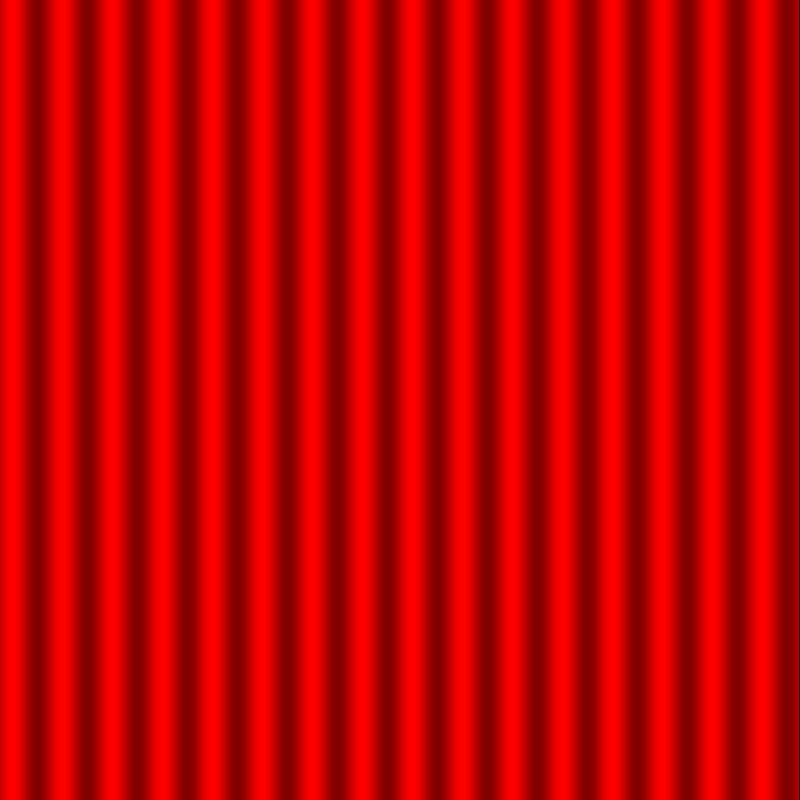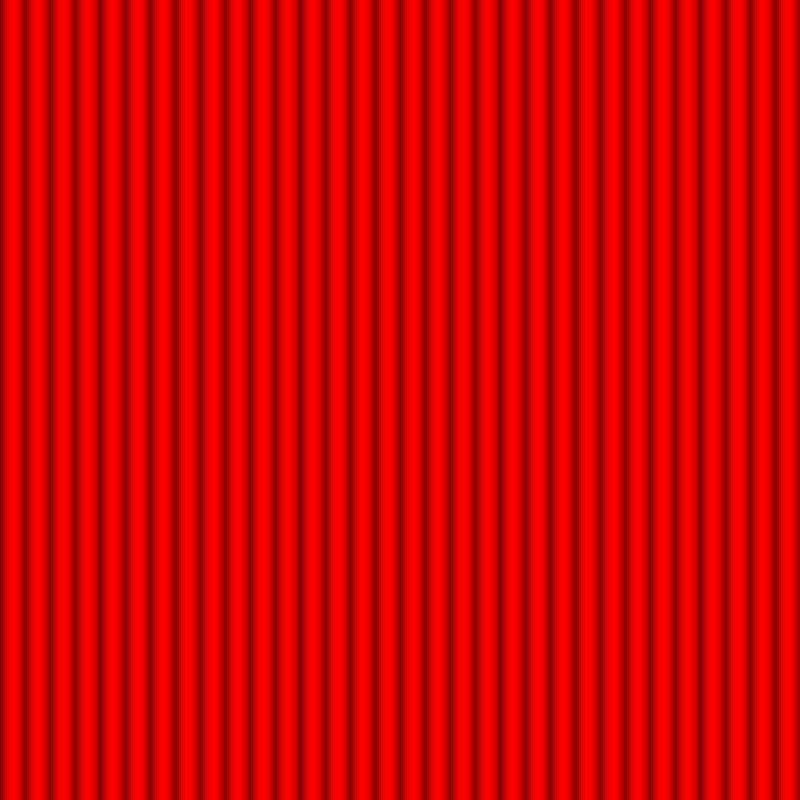# Yoga Mat

I own this yoga mat. The surface is textured, and I wanted to try to recreate it.

The approach: draw a bunch of (vertical) lines that vary in color. The main thing to vary here is the function to determine the line color, based on the x coordinate.

``````// Width of each "bar."
const SEGMENT_WIDTH = 30;
function draw() {
for (var x = 0; x < CANVAS_WIDTH; x++) {
r = // determine color
stroke(r, 0, 0);
line(x, 0, x, CANVAS_HEIGHT);
}
}
``````

To start, I tried sine:

``````// Normalize.
segmentX = (x % SEGMENT_WIDTH) / SEGMENT_WIDTH;
r = 255 * sin(TWO_PI * segmentX);
``````

The resulting image had a lot of black—that’s because the red value ranges from `[-255, 255]`. Mapping the range to `[128, 255]` gives a better image:

``````// Normalize.
segmentX = (x % SEGMENT_WIDTH) / SEGMENT_WIDTH;
r = map(sin(TWO_PI * segmentX), -1, 1, 128, 255);
``````The red shade here is “undulating,” but I wanted it to look more like “bouncing.” Taking the absolute value got what I wanted:

``````// Normalize.
segmentX = (x % SEGMENT_WIDTH) / SEGMENT_WIDTH;
r = map(abs(sin(TWO_PI * segmentX)), 0, 1, 128, 255);
``````This is essentially the yoga mat, but I wanted it to be a bit less uniform. This is handled by adding in noise when determining the shade of red:

``````segmentX = (x % SEGMENT_WIDTH) / SEGMENT_WIDTH;
r = map(abs(sin(TWO_PI * segmentX)), 0, 1, 128, 224)
+ map(noise(x/SEGMENT_WIDTH), 0, 1, 0, 32);
``````

After tweaking constants, I got an image I liked:Sketch available here.